# physics ch 7 Flashcards

Set Details Share
created 7 years ago by Elena_Kei
16,891 views
Page to share:
Embed this setcancel
COPY
code changes based on your size selection
Size:
X

1

1) You swing a bat and hit a heavy box with a force of 1500 N. The force the box exerts on the bat
is
A) exactly 1500 N only if the box does not move.
B) exactly 1500 N whether or not the box moves.
C) greater than 1500 N if the box moves.
D) less than 1500 N if the box moves.
E) greater than 1500 N if the bat bounces back.

B

2

2) In order to get an object moving, you must push harder on it than it pushes back on you.
A) True
B) False

B

3

3) In order to lift a bucket of concrete, you must pull up harder on the bucket than it pulls down
on you.
A) True
B) False

B

4

4) Consider what happens when you jump up in the air. Which of the following is the most
accurate statement?
A) It is the upward force exerted by the ground that pushes you up, but this force cannot
B) You are able to spring up because the earth exerts a force upward on you that is greater
than the downward force you exert on the earth.
C) Since the ground is stationary, it cannot exert the upward force necessary to propel you
into the air. Instead, it is the internal forces of your muscles acting on your body itself
that propels your body into the air.
D) When you push down on the earth with a force greater than your weight, the earth will
push back with the same magnitude force and thus propel you into the air.
E) When you jump up the earth exerts a force F1 on you and you exert a force F2 on the
earth. You go up because F1 > F2.

D

5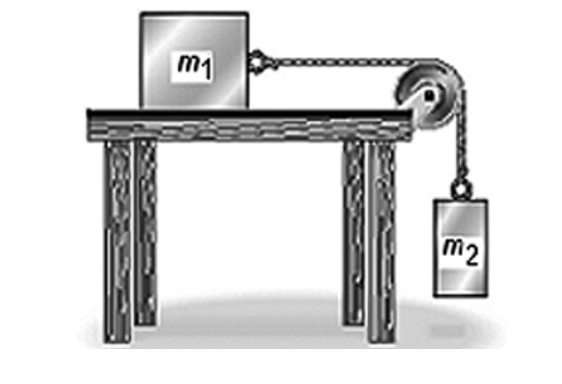7) Two objects having masses m1 and m2 are connected to each other as shown in the figure and
are released from rest. There is no friction on the table surface or in the pulley. The masses of
the pulley and the string connecting the objects are completely negligible. What must be true
about the tension T in the string just after the objects are released?

A) T = m2g
B) T > m2g
C) T < m2g
D) T = m1g
E) T > m1g

C

6

5) A 20‐ton truck collides with a 1500‐lb car and causes a lot of damage to the car. During the
collision
A) the force on the truck due to the collision is slightly greater than the force on the car.
B) the force of on the truck due to the collision is exactly equal to the force on the car.
C) the force on the car due to the collision is much greater than the force on the truck.
D) the car and the truck have the same magnitude acceleration.

B

7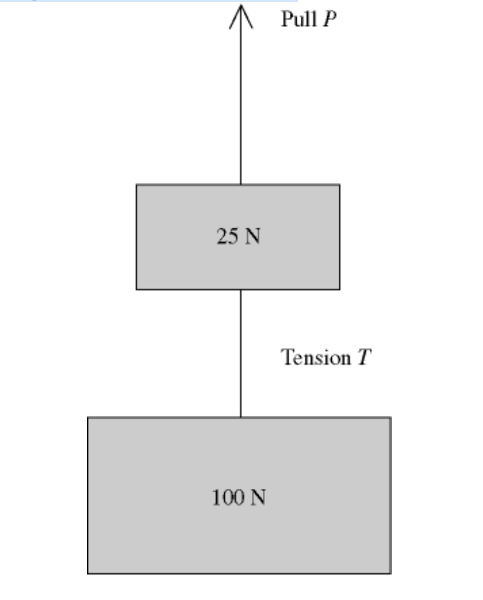6) Two weights are connected by a massless wire and pulled upward with a constant speed of
1.50 m/s by a vertical pull P. The tension in the wire is T (see figure). Which one of the
following relationships between T and P must be true?

A) T > P
B) T = P
C) P + T = 125 N
D) P = T + 25 N
E) P = T + 100 N

D

8Two bodies P and Q on a smooth horizontal surface are connected by a light cord. The mass of
P is greater than that of Q. A horizontal force F (of magnitude F) is applied to Q as shown in
the figure, accelerating the bodies to the right. The magnitude of the force exerted by the
connecting cord on body P will be

A) zero.
B) less than F but not zero.
C) equal to F.
D) greater than F.

B

9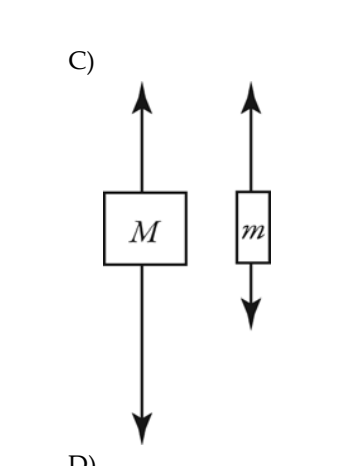9) Two unequal masses M and m (M > m) are connected by a light cord passing over a pulley of
negligible mass, as shown in the figure. When released, the system accelerates. Friction is
negligible. Which figure below gives the correct free‐body force diagrams for the two masses
in the moving system?

C

10

1) The International Space Station has a mass of 1.8 × 105 kg. A 70.0-kg astronaut inside the
station pushes off one wall of the station so she accelerates at 1.50 m/s2. What is the
magnitude of the acceleration of the space station as the astronaut is pushing off the wall?
Give your answer relative to an observer who is space walking and therefore does not
accelerate with the space station due to the push.
A) 5.8 × 10-4 m/s2
B) 1.50 m/s2
C) 4.7 × 10-4 m/s2
D) zero
E) 3.9 × 10-3 m/s2

A

11

On a horizontal frictionless floor, a worker of weight 0.900 kN pushes horizontally with a force
of 0.200 kN on a box weighing 1.80 kN. As a result of this push, which statement could be
true?
A) The box will not move because the push is less than its weight.
B) The worker and box will both have an acceleration of 1.08 m/s2, but in opposite
directions.
C) The worker and box will both have an acceleration of 2.17 m/s2, but in opposite
directions.
D) The worker will accelerate at 1.08 m/s2 and the box will accelerate at 2.17 m/s2, but in
opposite directions.
E) The worker will accelerate at 2.17 m/s2 and the box will accelerate at 1.08 m/s2, but in
opposite directions.

E

12

3) In a ballistics test, a 1.50-g bullet is fired through a 28.0-kg block traveling horizontally
toward the bullet. In this test, the bullet takes 11.4 ms to pass through the block as it reverses
the blockʹs velocity from 1.75 m/s to the right to 1.20 m/s to the left with constant acceleration.
Find the magnitude of the force that the bullet exerts on the block during this ballistics test.

13

4) A locomotive is pulling 19 freight cars, each of which is loaded with the same amount of
weight. The mass of each freight car (with its load) is 37,000 kg. If the train is accelerating at
0.22 m/s2 on a level track, what is the tension in the coupling between the second and third
cars? (The car nearest the locomotive is counted as the first car, and friction is negligible.)

14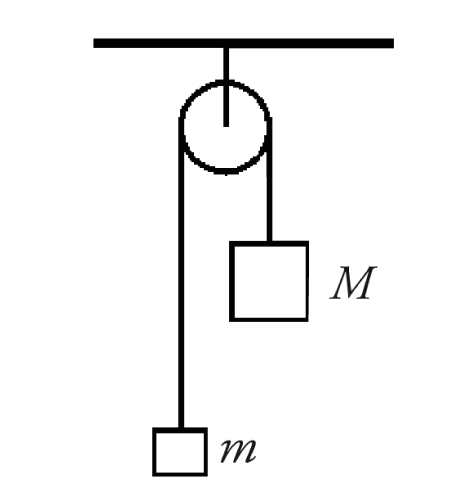5) Two objects are connected by a very light flexible string as shown in the figure, where M = 0.60
kg and m = 0.40 kg. You can ignore friction and the mass of the pulley.

(a) Draw free-body diagrams for each object.
(b) Calculate the magnitude of the acceleration of each object.
(c) Calculate the tension in the string.

(a) The force of gravity acts downward and tension acts upward on each object.
(b) 2.0 m/s2
(c) 4.7 N

15

6) Three boxes in contact rest side-by-side on a smooth, horizontal floor. Their masses are
5.0-kg, 3.0-kg, and 2.0-kg, with the 3.0-kg box in the center. A force of 50 N pushes on the
5.0-kg box, which pushes against the other two boxes.
(a) Draw the free-body diagrams for each of the boxes.
(b) What magnitude force does the 3.0-kg box exert on the 5.0-kg box?
(c) What magnitude force does the 3.0-kg box exert on the 2.0-kg box?

(a) The following forces act on the 5.0-kg box: the force due to gravity, normal force,
contact force between 5.0-kg mass and 3.0-kg mass, the force of 50 N pushing on the
box. The following forces act on the 3.0-kg box: the force due to gravity, normal force,
contact force between the 5.0-kg box and the 3.0-kg box, the contact force between the
3.0-kg box and the 2.0-kg box. The following forces act on the 2.0-kg box: the force
due to gravity, normal force, contact force between the 3.0-kg box and the 2.0-kg box.
(b) 25 N
(c) 10 N

16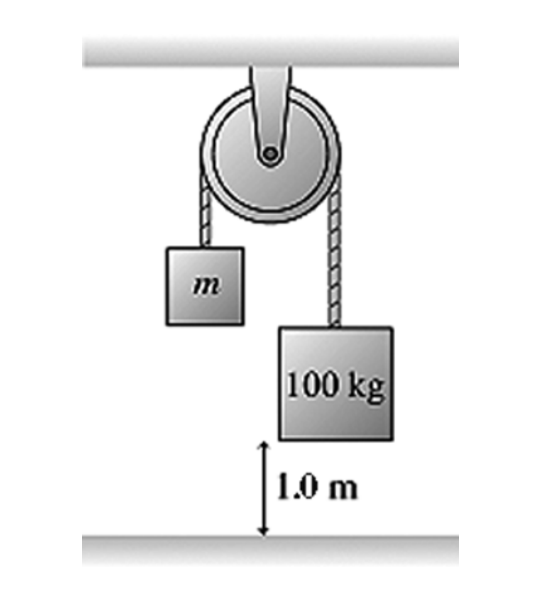The figure shows a 100-kg block being released from rest from a height of 1.0 m. It then takes
it 0.90 s to reach the floor. What is the mass m of the other block? The pulley has no
appreciable mass or friction.

A) 60 kg
B) 54 kg
C) 48 kg
D) 42 kg

A

17

A wooden block A of mass 4.0 kg slides on a frictionless table when pulled using a massless
string and pulley array by a hanging box B of mass 5.0 kg, as shown in the figure. What is the
acceleration of block A as it slides on the frictionless table? Hint: Think carefully about the
acceleration constraint.

A) 4.1 m/s2
B) 3.5 m/s2
C) 3.1 m/s2
D) 2.7 m/s2

A

18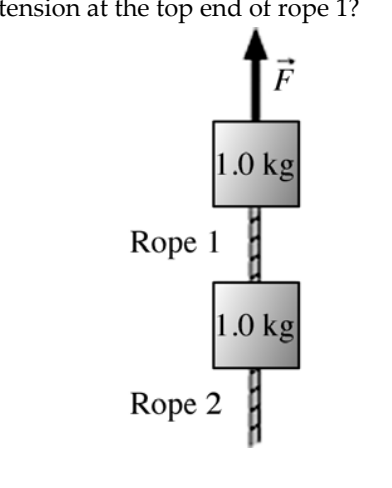The figure shows two 1.0 kg-blocks connected by a rope. A second rope hangs beneath the
lower block. Both ropes have a mass of 250 g. The entire assembly is accelerated upward at 2.3
m/s2 by force F . What is the tension at the top end of rope 1?

A) 18 N
B) 15 N
C) 2.9 N
D) 3.5 N

A

19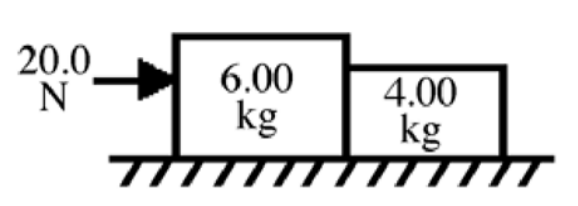A 6.00-kg block is in contact with a 4.00-kg block on a horizontal frictionless surface as shown
in the figure. The 6.00-kg block is being pushed by a horizontal 20.0-N force as shown. What
is the magnitude of the force that the 6.00-kg block exerts on the 4.00-kg block?

A) 6.00 N
B) 20.0 N
C) 8.00 N
D) 4.00 N
E) 10.0 N

C

20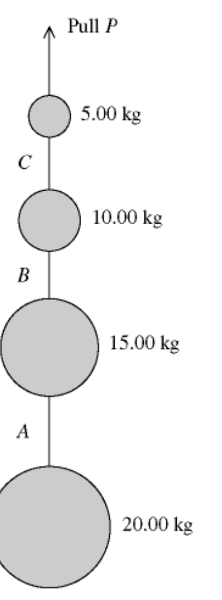A series of weights connected by very light cords are given an upward acceleration of 4.00
m/s2 by a pull P, as shown in the figure. A, B, and C are the tensions in the connecting cords.
The pull P is closest to

A) 690 N.
B) 490 N.
C) 290 N.
D) 200 N.
E) 50 N.

A

21A series of weights connected by very light cords are given an upward acceleration of 4.00
m/s2 by a pull P, as shown in the figure. A, B, and C are the tensions in the connecting cords.
The SMALLEST of the three tensions, A, B, and C, is closest to

C

22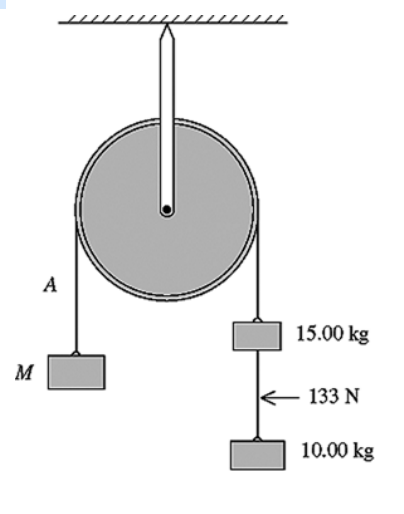Three objects are connected by massless wires over a massless frictionless pulley as shown in
the figure. The tension in the wire connecting the 10.0-kg and 15.0-kg objects is measured to
be 133 N. What is the mass M?

A) 8.33 kg
B) 33.9 kg
C) 35.0 kg
D) 52.8 kg
E) 95.0 kg

D

23Three objects are connected by massless wires over a massless frictionless pulley as shown in
the figure. The tension in the wire connecting the 10.0-kg and 15.0-kg objects is measured to
be 133 N. What is the tension in wire A?

A) 87.5 N
B) 245 N
C) 280 N
D) 333 N
E) 517 N

D

24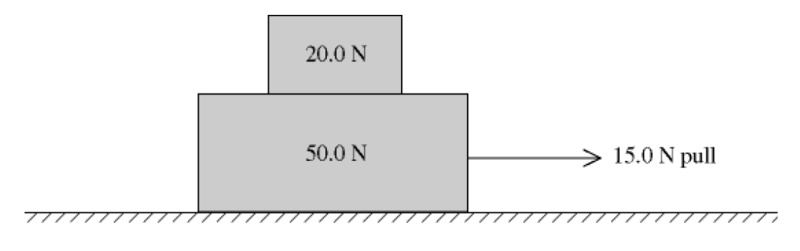15) A 20.0-N box rests on a 50.0-N box on a perfectly smooth horizontal floor. When a horizontal
15.0-N pull to the right is exerted on the lower box (see figure), both boxes move together. Find
the magnitude and direction of the net external force on the upper box.

4.29 N to the right

25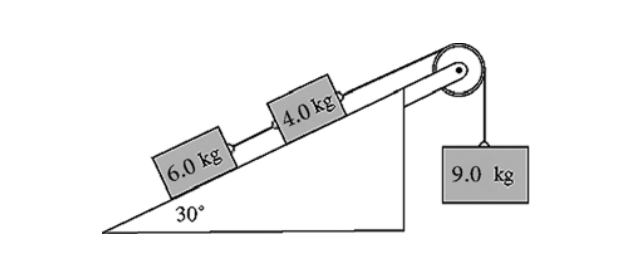A system comprising blocks, a light frictionless pulley, a frictionless incline, and connecting
ropes is shown in the figure. The 9.0-kg block accelerates downward when the system is
released from rest. The tension in the rope connecting the 6.0-kg block and the 4.0-kg block is
closest to

A) 30 N.
B) 33 N.
C) 36 N.
D) 39 N.
E) 42 N.

E

26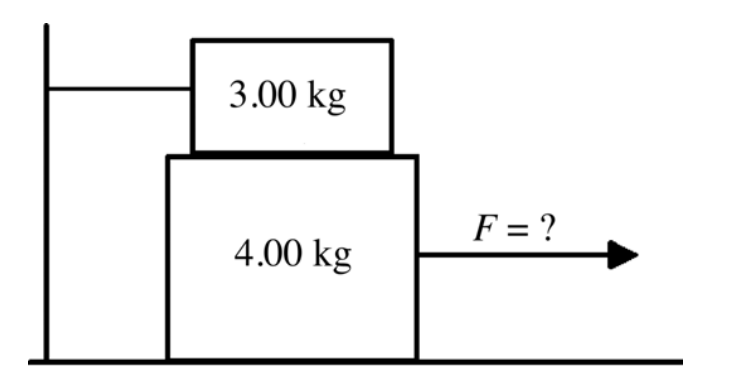17) A 4.00-kg block rests between the floor and a 3.00-kg block as shown in the figure. The
3.00-kg block is tied to a wall by a horizontal rope. If the coefficient of static friction is 0.800
between each pair of surfaces in contact, what horizontal force F must be applied to the
4.00-kg block to make it move?

A) 16.2 N
B) 54.9 N
C) 21.1 N
D) 23.5 N
E) 78.4 N

E

27

A 150-N box is being pulled horizontally in a wagon accelerating uniformly at 3.00 m/s2. The
box does not move relative to the wagon, the coefficient of static friction between the box and
the wagonʹs surface is 0.600, and the coefficient of kinetic friction is 0.400. The friction force on
this box is closest to
A) 450 N.
B) 90.0 N.
C) 60.0 N.
D) 45.9 N.

D

28

A pickup truck is moving at 25 m/s with a toolbox of mass m resting on the bed of the truck 2.5
m behind the cab. Suddenly the brakes are applied, causing the toolbox to slide, and the truck
comes to a stop in 4.7 s. The coefficient of kinetic friction between the toolbox and the bed of
the truck is 0.28. After the brakes are applied, how much time elapses before the toolbox
strikes the cab?

1.4

29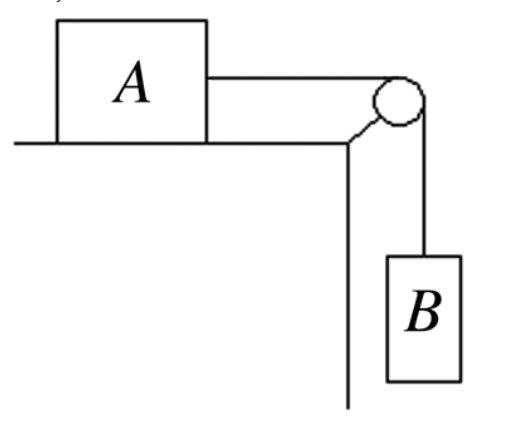Two boxes are connected by a weightless cord running over a very light frictionless pulley as
shown in the figure. Box A, of mass 8.0 kg, is initially at rest on the top of the table. The
coefficient of kinetic friction between box A and the table is 0.10. Box B has a mass of 15.0 kg,
and the system begins to move just after it is released.

(a) Draw the free-body diagrams for each of the boxes, identifying all of the forces acting on
each one.
(b) Calculate the acceleration of each box.
(c) What is the tension in the cord?

Answer: (a) Box A is acted on by the downward force of gravity, the upward normal force due to
the table top, the tension in the string toward the right, and the force of friction due to
the table top toward the left. Box B is acted on by the downward force of gravity and
the upward tension of the string.
(b) 6.1 m/s2
(c) 56 N

30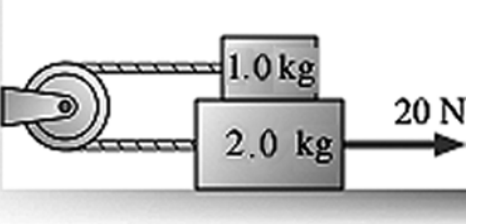A rope pulls on the lower block in the figure with a tension force of 20 N. The coefficient of
kinetic friction between the lower block and the surface is 0.16. The coefficient of kinetic
friction between the lower block and the upper block is also 0.16. The pulley has no
appreciable mass or friction. What is the acceleration of the 2.0 kg block?

A) 4.1 m/s2
B) 5.1 m/s2
C) 8.4 m/s2
D) 9.2 m/s2

A

31

Three objects are connected as shown in the figure. The strings and frictionless pulleys have
negligible masses, and the coefficient of kinetic friction between the 2.0-kg block and the table
is 0.25. What is the acceleration of the 2.0-kg block?

A) 2.5 m/s2
B) 1.7 m/s2
C) 3.2 m/s2
D) 4.0 m/s2

A

32

A system comprised blocks, a light frictionless pulley, and connecting ropes is shown in the
figure. The 9.0-kg block is on a perfectly smooth horizontal table. The surfaces of the 12-kg
block are rough, with μk = 0.30 between the block and the table. If the 5.0-kg block accelerates
downward when it is released, find its acceleration.

A) 1.0 m/s2
B) 1.2 m/s2
C) 1.4 m/s2
D) 1.6 m/s2
E) 1.8 m/s2

A

33

24) Block A of mass 5.0 kg and block X are attached to a rope which passes over a pulley, as
shown in the figure. An 80-N force P is applied horizontally to block A, keeping it in contact
with a rough vertical face. The coefficients of static and kinetic friction between the wall and
block A are μs = 0.40 and μk = 0.30. The pulley is light and frictionless. The mass of block X is
adjusted until block A moves upward with an acceleration of 1.6 m/s2. What is the mass of
block X?

A) 9.9 kg
B) 9.3 kg
C) 8.7 kg
D) 8.1 kg
E) 7.5 kg

a

34

In the figure, two wooden blocks each of 0.30 kg mass are connected by a string that passes
over a very light frictionless pulley. One block slides on a horizontal table, while the other
hangs suspended by the string, as shown in the figure. At time t = 0, the suspended block is
0.80 m over the floor, and the blocks are released from rest. After 2.5 s, the suspended block
reaches the floor. What is the coefficient of kinetic friction between the table and the sliding
block?

A) 0.35
B) 0.52
C) 0.84
D) 0.65
E) 0.95

E

35

26) A 4.00-kg box sits atop a 10.0-kg box on a horizontal table. The coefficient of kinetic friction
between the two boxes and between the lower box and the table is 0.600, while the coefficient
of static friction between these same surfaces is 0.800. A horizontal pull to the right is exerted
on the lower box, as shown in the figure, and the boxes move together. What is the friction
force on the UPPER box?

A) 19.3 N to the right
B) 19.3 N to the left
C) 23.5 N to the right
D) 31.4 N to the right
E) 31.4 N to the left

a

36

27) Two blocks are connected by a string that goes over an ideal pulley as shown in the figure.
Block A has a mass of 3.00 kg and can slide over a rough plane inclined 30.0° to the horizontal.
The coefficient of kinetic friction between block A and the plane is 0.400. Block B has a mass of
2.77 kg. What is the acceleration of the blocks?

A) 0.392 m/s2
B) 1.96 m/s2
C) 3.12 m/s2
D) 5.35 m/s2
E) 0.00 m/s2

a

37

28) The figure shows two packages that start sliding down a 20° ramp from rest a distance
d = 6.6 m along the ramp from the bottom. Package A has a mass of 5.0 kg and a coefficient of
kinetic friction 0.20 between it and the ramp. Package B has a mass of 10 kg and a coefficient of
kinetic friction 0.15 between it and the ramp. How long does it take package A to reach the
bottom?

A) 2.7 s
B) 3.0 s
C) 3.2 s
D) 3.5 s

a

38

29) Two weights are connected by a massless wire and pulled upward with a constant speed of
1.50 m/s by a vertical pull P. The tension in the wire is T (see figure). P is closest to

A) 25 N.
B) 125 N.
C) 187.5 N.
D) 245 N.
E) 1225 N.

b

39

30) Two blocks are connected by a string that goes over an ideal pulley as shown in the figure and
pulls on block A parallel to the surface of the plane. Block A has a mass of 3.00 kg and can slide
along a rough plane inclined 30.0° to the horizontal. The coefficient of static friction between
block A and the plane is 0.400. What mass should block B have in order to start block A sliding
up the plane?

2.54

40

The figure shows a 2000 kg cable car descending a high hill. A counterweight of mass 1800 kg
on the other side of the hill aids the brakes in controlling the cable carʹs speed. The rolling
friction of both the cable car and the counterweight are negligible. How much braking force
does the cable car need to descend at constant speed?

A) 3800 N
B) 2900 N
C) 2000 N
D) 980 N

A

41

32) A system of blocks and a frictionless pulley is shown in the figure. Block A has a mass of 2.0 kg
and is on a rough horizontal surface for which μs = 0.40 between the surface and block A. The
rope pulls horizontally on block A. Block C has a mass of 1.0 kg. An external force P = 23.0 N,
applied vertically to block A, maintains the system at rest as shown in the figure. What is the
friction force on block A?

A) 6.3 N
B) 6.9 N
C) 7.5 N
D) 5.7 N
E) 5.1 N

A

42

33) A 1520-N crate is to be held in place on a ramp that rises at 30.0° above the horizontal (see
figure). The massless rope attached to the crate makes a 22.0° angle above the surface of the
ramp. The coefficients of friction between the crate and the surface of the ramp are μk = 0.450
and μs = 0.650. The pulley has no appreciable mass or friction. What is the MAXIMUM weight
w that can be used to hold this crate stationary on the ramp?

Var: 1

43

34) Block A of mass 8.0 kg and block X are attached to a rope that passes over a pulley. A 50-N
force P is applied horizontally to block A, keeping it in contact with a rough vertical face. The
coefficients of static and kinetic friction between the wall and block A are μs = 0.40 and μk =
0.30. The pulley is light and frictionless. In the figure, the mass of block X is adjusted until
block A descends at constant velocity of 4.75 cm/s when it is set into motion. What is the mass
of block X?

A) 6.5 kg
B) 7.2 kg
C) 8.0 kg
D) 8.8 kg
E) 9.5 kg

A